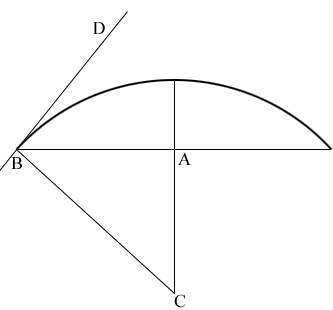SEARCH HOMEMath Central Quandaries & QueriesQuestion from nuel, a student: Hi, Is there any formula to calculate the arc length from only the chord length and angle between the chord and tangent of the arc at its endpoint ? Thank you.Nuel,

In my diagram $C$ is the centre of the circle, $A$ is the midpoint of the chord and $D$ is a point on the tangent line.Angle $DBC$ is a right angle and you know the measure of angle $DBA$ and hence in the right triangle $ABC$ you know the measure of the angle $ABC$ and the length of $AB.$ Can you complete the problem from here?

PennyMath Central is supported by the University of Regina and The Pacific Institute for the Mathematical Sciences.